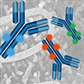# Product Results## Primary Antibody Conjugates

Choose from a wide range of directly conjugated primary antibodies, validated and optimized for your specific application. Not seeing the one you want? Let CST conjugate it for you.

# Product Name Application Reactivity
H, M, R, Mk, Dm, Z
H, M, R, Hm, Mk, Dm, Z, B
H, M, R, Hm, Mk, Dm, Z, B
H, M, R, Hm, Mk, Dm, Z, B
H, M, R, Hm, Mk, Dm, Z, B
H, M, R, Hm, Mk, Dm, Z, B
H, M, R, Hm, Mk, Dm, Z, B
H, M, R, Hm, Mk, Dm, Z, B
H, R, Mk, Z, B, Pg
H, R, Mk, Z, B, Pg
H, R, Mk, Z, B, Pg
H, R, Mk, Z, B, Pg
H, M, R, Mk, Z, Dg
H, M, R, Mk, Z, Dg
H, M, R, Hm, Mk, Mi, Dm, Z, B, Dg, Pg, Sc
H, M, R, Mk, Mi, Dm, Z, Pg
H, M, R, Hm, Mk, Mi, Z, B, Pg
H, M, R, Mk, Mi, Dm, Z, Pg
H, M, R, Mk, Mi, Dm, Z, Pg
H, M, R, Mk, Mi, Dm, Z, Pg
H, M, R, Hm, Mk, Mi, Z, B, Pg
H, M, R, Mk, Mi, Dm, Z, Pg
H, M, R, Mk, Mi, Dm, Z, Pg
H, M, R, Mk, Mi, Dm, Z, Pg
H, M, R, Mk, Mi, Dm, Z, Pg
H, M, R, Mk, Mi, Dm, Z, Pg
H, M, R, Hm, Mk, Mi, Z, B, Pg
H, M, R, Hm, Mk, Mi, Dm, Z, B, Dg, Pg, Ce
H, M, R, Hm, Mk, Mi, Dm, Z, B, Dg, Pg, Ce
H, M, R, Hm, Mk, Mi, Dm, Z, B, Dg, Pg, Ce